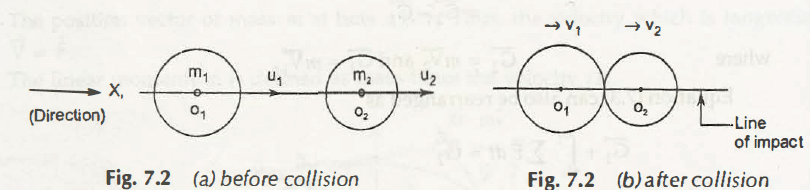Direct Collision is also termed as one-dimensional collisions or head-on collision. This is because both the bodies have same line of impact before collision.

Let us consider two bodies of mass m1 and m2 respectively

Initial Velocities are u1 and u2respectively in x-direction as shown in Fig. 7.2 (a)

After collision, the velocities of the bodies change to v1 and v2respectively in x-direction as shown in Fig. 7.2 (b)Total momentum before collision= m1u1 + m2u2

Total momentum after collision= m1v1 + m2v2

According to law of conservation of momentum, total momentum can be written as-

m1u1 + m2u2 = m1v1 + m2v2